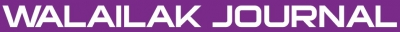### Numerical Solution of the Uncertain Characteristic Cauchy Reaction-Diffusion Equation by Variational Iteration Method

Hamid ROUHPARVAR, Saeid ABBASBANDY

#### Abstract

In this paper, the uncertain characteristic Cauchy reaction-diffusion equation is solved by the variational iteration method. The uncertain characteristic Cauchy problem is converted to a system of characteristic Cauchy problems by parametric representation and LU-fuzzy representation of fuzzy numbers. Also, using the variational iteration method and 2 representations of fuzzy numbers, 2 representations of approximate fuzzy solution are found and compared. Finally, the method is explained by 2 illustrative examples.

doi:10.14456/WJST.2014.73

#### Keywords

Uncertain characteristic Cauchy problem, LU-fuzzy representation, parametric representation, variational iteration method

PDF

#### References

D Dubois and H Prade. Operations on fuzzy numbers. J. Systems Sci. 1978; 9, 613-26.

S Nahmias. Fuzzy variables. Fuzzy Set. Syst. 1989; 32, 95-101.

M Mizumoto and K Tanaka. The four operations of arithmetic on fuzzy numbers. Syst. Comput. Contr. 1976; 7, 73-81.

R Goetschel and W Voxman. Elementery calculus. Fuzzy Set. Syst. 1986; 18, 31-43.

E Babolian, HS Goghary and S Abbasbandy. Numerical solution of linear Fredholm fuzzy integral equations of the second kind by Adomian method. Appl. Math. Comput. 2005; 161, 733-44.

T Allahviranloo, N Ahmady and E Ahmady. Numerical solution of fuzzy differential equations by predictor-corrector method. Inform. Sci. 2007; 177, 1633-47.

S Seikkala. On the fuzzy initial value problem. Fuzzy Set. Syst. 1987; 24, 319-30.

P Diamond and PE Kloeden. Metric Spaces of Fuzzy Sets: Theory and Applications. World Scientific, Singapore, 1994.

L Stefanini. Differential Evolution Methods for the Fuzzy Extension of Functions. Working Paper Series EMS, University of Urbino, Urbino, Italy, 2006.

L Stefanini. Generalized LU-Fuzzy Derivative and Numerical Solutions of Fuzzy Differential Equations. Working Paper Series EMS, University of Urbino, Urbino, Italy, 2007.

L Stefanini, L Sorinia and ML Guerra. Parametric representation of fuzzy numbers and application to fuzzy calculus. Fuzzy Set. Syst. 2006; 157, 2423-55.

M Dehghan and F Shakeri. Application of He’s variational iteration method for solving the Cauchy reaction-diffusion problem. J. Comput. Appl. Math. 2008; 214, 435-46.

MA Noor and ST Mohyud-Din. Variational iteration technique for solving higher order boundary value problems. App. Math. Comput. 2007; 189, 1929-42.

JH He and XH Wu. Construction of solitary solution and compacton-like solution by variational iteration method. Chaos Soliton. Fract. 2006; 29, 108-13.

NH Sweilam and MM Khader. Variational iteration method for one dimensional nonlinear thermo-elasticity. Chaos Soliton. Fract. 2007; 32, 145-9.

NH Sweilam and MM Khader. On the convergence of variational iteration method for nonlinear coupled system of partial differential equations. Int. J. Comp. Math. 2010; 87, 1120-30.

ZM Odibat. A study on the convergence of variational iteration method. Math. Comput. Modell. 2010, 51, 1181-92.

M Tatari and M Dehghan. On the convergence of He’s variational iteration method. J. Comput. Appl. Math. 2007; 207, 121-8.

NF Britton. Reaction-Diffusion Equations and Their Applications to Biology. Harcourt Brace Jovanovich Publishers, NewYork, 1998.

RS Cantrell and C Cosner. Spatial Ecology via Reaction-Diffusion Equations. Wiley, Chichester, 2003.

J Smoller. Shock Waves and Reaction-Diffusion Equations Grundlehren der Mathematischen Wissenschaften (Fundamental Principles of Mathematical Sciences). 2nd ed. Springer, New York, 1994.

G Wang and C Wu. Directional derivatives and subdifferential of convex fuzzy mappings and application in convex fuzzy programming. Fuzzy Set. Syst. 2003; 138, 559-91.

JH He. Approximate analytical solution for seepage flow with fractional derivatives in porous media. Comput. Meth. Appl. Mech. Eng. 1998; 167, 57-68.

JH He. Approximate Analytical Methods in Science and Engineering (in Chinese). Henan Science and Technology Press, Zhengzhou, 2002.

### Refbacks

• There are currently no refbacks.Online ISSN: 2228-835X

http://wjst.wu.ac.th

Last updated: 20 June 2019